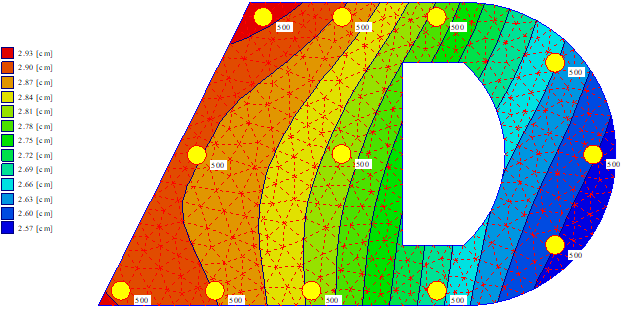Besides the following tutorial and verification examples, ELPLA solves different civil engineering problems.
• Soil-structure interaction problems.
• Analysis and design of rafts
• Analysis of rigid rafts
• Analysis of flexible foundations
• Analysis and design of slab floors
• Determining the consolidation settlements
• Analysis and design of pile caps
• Determining forces on piles due to structure loads
• Settlement calculation of surface foundations
• Determining the settlement due to surcharge fills or surcharge concentrated loads
• Determining the surface settlement around rafts
• Determining the constant or variable modulus of subgrade reaction
• Effect of external loads or neighboring foundations
• Effect of temperature difference
• Effect of tunneling
• Analysis of system of flexible, elastic, or rigid foundations
• Analysis of beams or grids by FE-Method
• Simulation of excavations and construction of embankments
• Determining the ultimate bearing capacity of the soil
• Determining the limit depth
• Eliminating negative contact pressure
• Design of slabs, rafts, and piled rafts according to codes ACI, EC 2, DIN 1045, and ECP
• Determining stresses, strains, and displacements in soil
• Analysis of plane frame
• Analysis of plane stress
• Analysis of grid
• Analysis of a system of many slab foundations
• Analysis of rotational shell
• Analysis of axisymmetric stress
• Analysis of axisymmetric structures
• Analysis of cylindrical tanks
• Analysis of beam on elastic foundation
• Analysis of grid on elastic foundation
• Dynamic analysis of beams
• Dynamic analysis of trusses
• Dynamic analysis of grids
• Dynamic analysis of space frames
• Dynamic analysis of shear walls
• Dynamic analysis of floor slabs
• Dynamic analysis of axisymmetric solids

### Subcategories

This Tutorial Manual contains an overview of dealing with structural problems such as raft, piled raft, pile group, a system of rafts, slab floor, grid, truss, beam, plane frame, and plane stress. It describes the processes of modeling the problems, carrying out the analyses, viewing and printing the results. It provides the user skills, which he needs to use ELPLA. It also takes the user gradually through some simple examples. Carrying out these examples will help the user to become familiar with the most important functions of ELPLA. Before attempting a real project with ELPLA, it is recommended that the user tries to carry out the given problems.

This Tutorial Manual will not present the theoretical background of modeling the problems. For more information concerning the methods of analysis, a complete reference for calculation methods and numerical models is well documented in the User’s Guide of ELPLA. In addition, a complete reference for all menus and dialog boxes of the program is to be found in the User’s Guide or in the online help system.

The usage of the program is typically such that first data files are created describing a certain problem through the "Data" Tab. Then the project problem is analyzed by calculation commands through the "Solver" Tab. Finally, results can be presented as graphical drawings, graphs, and tables through the "Result" Tab.

This Tutorial contains 13 examples. For each example discussed in this Tutorial, data files and some computed files are included in ELPLA software package. The file names and contents of the examples are given as follows. Besides, a key figure of each problem is shown that contains the main data concerning the structure shape and loads. Examples can be run again by ELPLA to examine the details of the analysis or to see how the problem was defined or computed and to display, print, or plot the results.